Students can download 10th Science Chapter 9 Solutions Questions and Answers, Notes, Samacheer Kalvi 10th Science Guide Pdf helps you to revise the complete Tamilnadu State Board New Syllabus, helps students complete homework assignments and to score high marks in board exams.

## Tamilnadu Samacheer Kalvi 10th Science Solutions Chapter 9 Solutions

### Samacheer Kalvi 10th Science Solutions Text Book Back Questions and Answers

Question 1.
A solution is a mixture.
(a) homogeneous
(b) heterogeneous
(c) homogeneous and heterogeneous
(d) non-homogeneous
(a) homogeneous

Question 2.
The number of components in a binary solution is ______.
(a) 2
(b) 3
(c) 4
(d) 5.
(a) 2

Question 3.
Which of the following is the universal solvent?
(a) Acetone
(b) Benzene
(c) Water
(d) Alcohol
(c) WaterQuestion 4.
A solution in which no more solute can be dissolved in a definite amount of solvent at a given temperature is called ______.
(a) Saturated solution
(b) Un saturated solution
(c) Supersaturated solution
(d) Dilute solution.
(a) Saturated solution

Question 5.
Identify the non-aqueous solution.
(a) sodium chloride in water
(b) glucose in water
(c) copper sulphate in water
(d) sulphur in carbon-di-sulphide
(d) sulphur in carbon-di-sulphide

Question 6.
When pressure is increased at a constant temperature the solubility of gases in liquid ______.
(a) No change
(b) increases
(c) decreases
(d) no reaction.
(b) increases

Question 7.
Solubility of NaCl in 100 ml water is 36 g. If 25 g of salt is dissolved in 100 ml of water how much more salt is required for saturation:
(a) 12 g
(b) 11 g
(c) 16 g
(d) 20 g
(b) 11 g

Question 8.
A 25% alcohol solution means ______.
(a) 25 ml of alcohol in. 100 ml of water
(b) 25 ml of alcohol in 25 ml of water
(c) 25 ml of alcohol in 75 ml of water
(d) 75 ml of alcohol in 25 ml of water.
(c) 25 ml of alcohol in 75 ml of water

Question 9.
Deliquescence is due to:
(a) Strong affinity to water
(b) Less affinity to water
(c) Strong hatred to water
(d) Inertness to water
(a) Strong affinity to water

Question 10.
Which of the following is hygroscopic in nature?
(a) ferric chloride
(b) copper sulphate pentahydrate
(c) silica gel
(d) none of the above.
(c) silica gel

II. Fill in the blanks:

1. The component present in lesser amount, in a solution is called ……..
2. Example for liquid in solid type solution is ……….
3. Solubility is the amount of solute dissolved in ……… g of solvent.
4. Polar compounds are soluble in ……… solvents.
5. Volume percentage decreases with increases in temperature because ………

1. solute
2. amalgam
3. 100
4. Polar
5. of expansion of liquid

III. Match the following: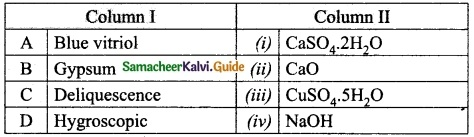A. (iii)
B. (i)
C. (iv)
D. (ii)IV. True or False: (If false give the correct statement):

1. Solutions which contain three components are called binary solution.
2. In a solution the component which is present in lesser amount is called solvent.
3. Sodium chloride dissolved in water forms a non-aqueous solution.
4. The molecular formula of green vitriol is MgSO4. 7H2O
5. When Silica gel is kept open, it absorbs moisture from the air, because it is hygroscopic in nature.

1. False – Solutions which contain two components are called binary solution.
2. False – In a solution the component which is present in lesser amount is called solute.
3. False – Sodium chloride dissolved in water forms an aqueous solution.
4. False – The molecular formula of green vitriol is FeSO4. 7H2O
5. True

Question 1.
Define the term: Solution
A solution is a homogeneous mixture of two or more substances.

Question 2.
What is mean by the binary solution?
A solution must at least be consisting of two components. Such solutions which are made of one solute and one solvent are called binary solutions.
E.g., On adding CuSO4 crystals to water.

Question 3.
Give an example each

1. gas in liquid;
2. solid in liquid;
3. solid in solid;
4. gas in gas.

1. Gas in liquid – CO2 in water
2. Solid in liquid – NaCl in water
3. Solid in solid – Alloys
4. Gas in gas – He – O2 gas

Question 4.
What is the aqueous and non-aqueous solution? Give an example.
Aqueous solution: The solution in which water act as a solvent is called aqueous solution. In general, ionic compounds are soluble in water and form aqueous solutions more readily than covalent compounds. E.g. Common salt in water.

Non – Aqueous solution: The solution in which any liquid, other than water act as a solvent is called non-aqueous solution. Alcohols, benzene, ethers, etc., are used as non – aqueous solvents. E.g. Sulphur dissolved in carbon disulphide.

Question 5.
Define Volume percentage.
Volume percentage is defined as the percentage by volume of solute (in ml) present in the given volume of solution.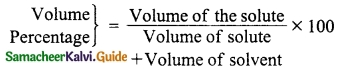Question 6.
The aquatic animals live more in a cold region. Why?
Aquatic animals live more in cold regions because the solubility of oxygen is more in cold water (at low temperature). Therefore, aquatic animals are more comfortable in cold water.

Question 7.
Define Hydrated salt.
Ionic substances which crystallise out from their saturated aqueous solution with a definite number of molecules of water are called hydrated salts.

Question 8.
A hot saturated solution of copper sulphate forms crystals as it cools. Why?
The capability of a solution to maintain a certain concentration of solute is temperature-dependent. When a saturated solution of copper sulphate at above room temperature is allowed to cool, the solution becomes supersaturated and in the absence of stirring or the return of the previous solution temperature, the solute starts to precipitate out. i.e., crystal formation occurs.Question 9.
Classify the following substances into deliquescent, hygroscopic. Cone. Sulphuric acid, Copper sulphate penta hydrate, Silica gel, Calcium chloride and Gypsum salt.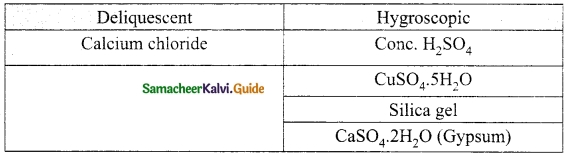Question 1.
Write notes on?

1. saturated solution
2. unsaturated solution

1. Saturated solution: A solution in which no more solute can be dissolved in a definite amount of the solvent at a given temperature is called saturated solution, e.g. 36 g of sodium chloride in 100 g of water at 25°C forms a saturated solution.
2. Unsaturated solution: Unsaturated solution is one that contains less solute than that of the saturated solution at a given temperature, e.g. 10 g or 20 g or 30 g of Sodium chloride in 100 g of water at 25°C forms an unsaturated solution.

Question 2.
Write notes on various factors affecting solubility.
There are three main factors which affects the solubility of a solute. They are

1. Nature of the solute and solvent
2. Temperature
3. Pressure

1. Nature of the solute and solvent : The nature of the solute and solvent plays an important role in solubility. Even though water is Universal solvent, all substances do not dissolve in water. Dissolution occurs when similarities exist between the solvent and the solute.

Ionic compounds are soluble in polar solvent like water and covalent compounds are soluble in non-polar solvents like ether, benzene, alcohol etc.

2. Effect of Temperature :
Solubility of solid in liquid : Generally solubility of a solid solute in a liquid increases with increase in temperature.
In Endothermic process : Solubility increases with increase in temperature.
In Exothermic process : Solubility decreases with increase in temperature.
Solubility of Gases in liquid : Solubility of gases in liquid decreases with increase in temperature.

3. Effect of Pressure : Effect of pressure is observed only in the case of solubility of a gas in a liquid. When the pressure is increased, the solubility of a gas in liquid increases.

Question 3.
(a) What happens when MgSO4.7H2O is heated? Write the appropriate equation
(b) Define solubility.
(a) When Epsom salt MgSO4.7H2O crystals are gently heated, it loses seven water molecules and becomes anhydrous MgSO4.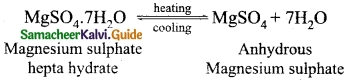(b) Solubility is defined as the amount of solute in grams that can be dissolved in 100 g of the solvent to form its saturated solution at a given temperature and pressure.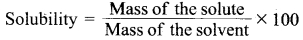Question 4.
In what way hygroscopic substances differ from deliquescent substances.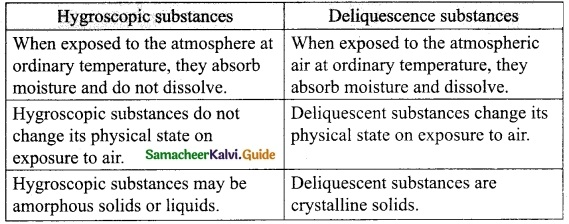Question 5.
A solution is prepared by dissolving 45 g of sugar in 180 g of water. Calculate the mass percentage of solute.
Mass of the solute (sugar) = 45 g
Mass of the solvent (Water) = 180 g
Formula:
Mass percentage of solute (sugar)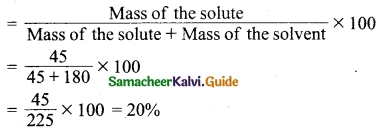The mass percentage of solute = 20%Question 6.
3.5 litres of ethanol is present in 15 litres of aqueous solution of ethanol. Calculate volume percent of ethanol solution.
Volume of ethanol = 3.5 lit = 3500 ml
Volume of water = 15 lit = 15000 ml
Formula: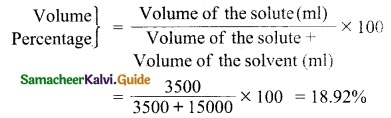The volume percentage of ethanol solution = 18.92

VII. HOT Questions

Question 1.
Vinu dissolves 50 g of sugar in 250 ml of hot water, Sarath dissolves 50 g of same sugar in 250 ml of cold water. Who will get a faster dissolution of sugar? and Why?
Vinu will get a faster dissolution of sugar. Because generally solubility of a solid solute in a liquid solvent increases with increase in temperature. Therefore Vinu dissolves 50 g of sugar in 250 ml of hot water than Sarath dissolves 50 g of sugar in 250 ml of cold water.

Question 2.
‘A’ is a blue coloured crystalline salt. On heating it loses blue colour and to give ‘B’ When water is added, ‘B’ gives back to ‘A’. Identify A and B, write the equation.
Since ‘A’ is a blue coloured crystalline salt, it is CuSO4. 5H2O (Blue vitriol). On heating it loses all five water molecules and becomes colourless anhydrous CuSO4.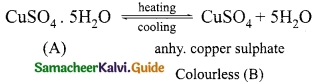When water is added ‘B’ gives back A.

Question 3.
Will the cool drinks give more fizz at top of the hills or at the foot? Explain.
At hilltops, the temperature will become less and pressure also decreases. Because temperature and pressure are directly proportional to each other. At low-pressure carbonate, cool drinks will give less fizz and give more fizz at the foot.

Question 1.
The dissolution of sugar and salt in water results in a solution.
(a) Binary
(b) Ternary
(c) Quaternary
(d) Saturated
(b) Ternary

Question 2.
In a solution, the component which is present in a lesser amount is called ______.
(a) solvent
(b) dissolution
(c) solute
(d) mole.
(c) solute

Question 3.
The supersaturated solution of NaCl in 100 g of water at 25°C contains:
(a) 40 g of NaCl
(b) 10 g of NaCl
(c) 20 g of NaCl
(d) 30 g of NaCl
(a) 40 g of NaClQuestion 4.
How many component(s) present in binary solution?
(a) 1
(b) 2
(c) 3
(d) 4.
(b) 2

Question 5.
Formalin is an aqueous solution of:
(a) formic acid
(b) ammonia
(c) formaldehyde
(d) carbon tetrachloride
(c) formaldehyde

Question 6.
The effect of pressure on the solubility of a gas in liquids is given by:
(a) Boyle’s Law
(b) Charle’s Law
(c) Henry’s Law
(c) Henry’s Law

Question 7.
Which one of the following is an example of an aqueous solution?
(a) Sugar in water
(b) Sulphur in carbon disulphide
(c) Iodine dissolved in carbon tetrachloride
(d) Benzoic acid in ethers.
(a) Sugar in water

Question 8.
The type of solution when CO2 is dissolved in water:
(a) solid/liquid
(b) liquid in liquid
(c) gas in liquid
(d) liquid in solid
(c) gas in liquid

Question 9.
Tin amalgam is an example of ……… solution.
(a) solid in solid
(b) liquid in solid
(c) solid in liquid
(d) liquid in liquid
(b) liquid in solid

Question 10.
In which case solubility decreases with increase in temperature?
(a) Endothermic process
(b) Exothermic process
(c) Both (a) and (b)
(d) None of these.
(b) Exothermic process

Question 11.
Fat is soluble in:
(a) water
(b) alcohol
(c) CCl4
(d) ether
(d) ether

Question 12.
The deliquescent substance among the following is:
(a) con.H2SO4
(b) P2O5
(c) CaCl2
(d) SiO2
(c) CaCl2Question 13.
Mass percentage is expressed as ______.
(a) v/v
(b) w/w
(c) v/w
(d) w/v.
(b) w/w

Question 14.
Hygroscopic substances are used as ……… agents.
(a) foaming
(b) drying
(c) oxidising
(d) reducing
(b) drying

Question 15.
The molecular formula of Epsom salt is ______.
(a) CuSO4.5H2O
(b) FeSO4.7H2O
(c) MgSO4.7H2O
(d) ZnSO4.7H2O.
(c) MgSO4.7H2O

II. Fill in the blanks:

1. A true solution is a ……… mixture of solute and solvent.
2. Soil cannot store more nitrogen than it can hold because soil is said to be in a state of ………
3. In the dissolution of NaOH in water, the solubility …….. with increase in temperature.
4. Aquatic animals are more comfortable in cold water because as the temperature is less the solubility of dissolved oxygen ………
5. Hydrated salts contain ……… of crystallization.
6. He-O2 mixture is a binary solution of …….. in ………. solution.
7. The solvent used for dissolving Sulphur is ……….
8. The solubility of NaOH at 25°C is ……….
9. According to Henry’s Law, the solubility of a gas in liquid is ………. proportional to the pressure of the gas over the solution at definite temperature.
10. Anhydrous Calcium chloride is a ………. substance.
11. ……… substances absorb enough water from the atmosphere and get completely dissolved.
12. When 90g of sodium bromide is dissolved in 100 g of water at 25°C it forms a ………. solution.
13. ………. is an example of a binary solution with liquid in Gas.
14. Air and sea water are important ……… solution.
15. A quaternary solution contains ……….. components.
16. The primary factor which determines the characteristic of a solution is ………..
1. Homogeneous
2. saturation
3. decreases
4. increases
5. water
6. Gas, Gas
7. CS2 (or) Carbon disulphide
8. 80 g
9. directly
10. Hygroscopic
11. deliquescent
12. Unsaturated
13. Cloud
14. Homogeneous
15. four
16. Physical stateIII. Match the following

Question 1.
Match the column I with column II.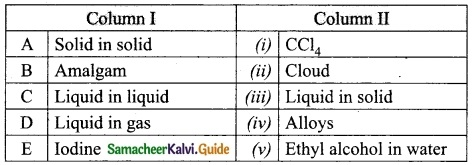A. (iv)
B. (iii)
C. (v)
D. (ii)
E. (i)

Question 2.
Match the column I with column II.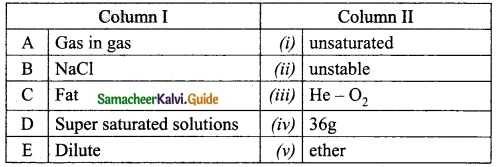A. (iii)
B. (iv)
C. (v)
D. (ii)
E. (i)

Question 3.
Match the column I with column II.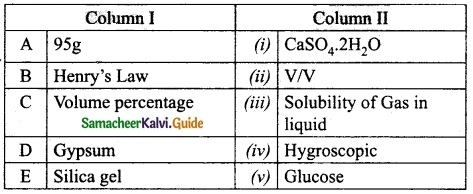A. (v)
B. (iii)
C. (ii)
D. (i)
E. (iv)

Question 4.
Match the column I with column II.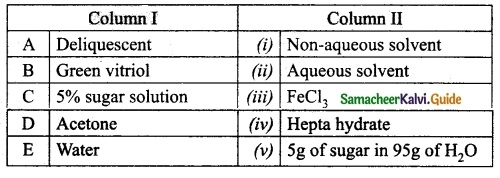A. (iii)
B. (iv)
C. (v)
D. (i)
E. (ii)

Question 5.
Match the column I with column II.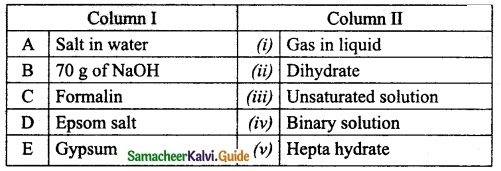A. (iv)
B. (iii)
C. (i)
D. (v)
E. (ii)

IV. True or False: (If false give the correct statement)

1. In an aqueous solution of copper sulphate, the solvent is copper sulphate.
2. A solution containing sugar and salt in water is a binary solution.
3. An example of a solid solution is alloy.
4. The difference between concentrated and dilute solution can be observed by means of colour (or) density.
5. A saturated solution contains 91 g of Glucose in 100 g of water at 25°C.
6. Fat is dissolved in the aqueous solvent ether.
7. The solubility of a gas in a liquid is inversely proportional to the pressure of the gas at a definite temperature.
8. Mass percentage of a solution is expressed as .
9. The white vitriol is represented by the formula ZnSO4 . 7H2O.
10. Ferric chloride is a Hygroscopic substance.
1. False – In an aqueous solution of copper sulphate, the solvent is water.
2. False – A solution containing sugar and salt in water is a ternary solution.
3. True
4. True
5. True
6. False – Fat is dissolved in the non-aqueous solvent ether.
7. False – The solubility of a gas in a liquid is directly proportional to the pressure of the gas at a definite temperature.
8. True
9. True
10. False – Ferric chloride is a deliquescent substance.Question 1.
(i) Which gas is dissolved in soft drinks?
(ii) What will you do to increase the solubility of this gas?
(i) Carbon-di-oxide (CO2) is dissolved in soft drinks.
(ii) An increase in pressure will increase the solubility of CO2 gas.

Question 2.
Identify the type of binary solution given below.

1. Alloys
2. Amalgam
3. Ethyl alcohol in water
4. Aerated drinks

1. Solid in solid
2. liquid in solid
3. liquid in liquid
4. Gas in liquid

Question 3.
Explain why Nitrogen in soil is called a saturated solution in nature?
Nitrogen in soil is an example of a saturated solution in nature. Soil cannot store more Nitrogen than it can hold.

Question 4.
Define Mass percentage.
Mass percentage of a solution is defined as the percentage by mass of the solute present in the solution.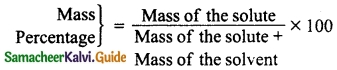Question 5.
Define the term Molarity (M).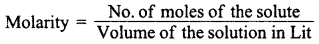Question 6.
Define the term Molality (m).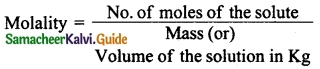Question 7.
Define the supersaturated solution.
A supersaturated solution is one that contains more solute than the saturated solution at a given temperature.
E.g. 40 g of sodium chloride in 100 g of water at 25°C.

Question 8.
Justify the following statements with an explanation.
(i) Solubility of NH4Cl increases with increase in temperature.
Solubility of NH4Cl increases with increase in temperature because it is an endothermic process.

(ii) Solubility of NaOH decreases with increase in temperature.
Solubility of NaOH decreases with increase in temperature because it is an exothermic process.

Question 9.
Calculate the molarity of a solution containing 4 g of NaOH in 500 ml of water.
Mass of NaOH = 4 g
Volume of solution = 500 ml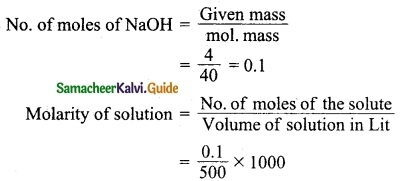= 0.1 × 2
= 0.2 M

Question 10.
Calculate the molality of a solution containing 3 g of urea (molecular mass = 60) in 750 g of water.
Mass of urea (solute) = 3 g
Mass of water (solvent) = 750 g
Formula: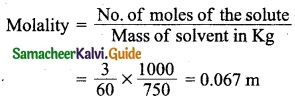Question 11.
Define dissolution.
The process of uniform distribution of solute into solvent is called dissolution.

Question 1.
Answer the blanks given in the table.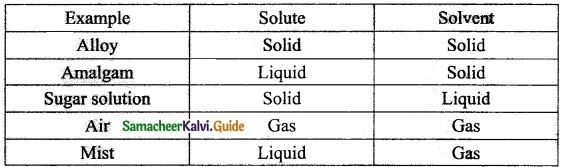Question 2.
Write a note on the type of solution based on the type of solvent.
(i) Aqueous solution : The solution in which water acts as a solvent is called aqueous solution. In general, ionic compounds are soluble in water and form aqueous solutions more readily than covalent compounds.
Eg: Common salt in water, Sugar in water, Copper sulphate in water etc..

(ii) Non-Aqueous solution : The solution in which any liquid, otter than water, acts as a solvent is called non-aqueous solution. Solvent other than water is referred to as non-aqueous solvent. Generally, alcohols, benzene, ethers, carbon disulphide, acetone, etc., are used as non- aqueous solvents.
Eg: Sulphur dissolved in carbon disulphide, Iodine dissolved in carbon tetrachloride.Question 3.
Justify the following statements with an explanation.

1. The solubility of calcium oxide decreases with increase in temperature,
2. What happens to the solubility in the exothermic process with regard to temperature?
3. In the endothermic process, solubility increases with increase in temperature.
4. At a given temperature, an increase in pressure increases the solubility of the gas

1. In an exothermic process, the solubility decreases. When calcium oxide dissolves in water, an exothermic reaction takes place, and so the solubility of calcium oxide decreases
2. In an exothermic process, the solubility decreases with the increase in temperature, as there is already an evolution of heat and it is observed.
3. In an endothermic process, the solubility increases. The solubility of KNO3 in water is an endothermic reaction and so solubility increases with the increase of temperature.
4. At a given temperature, an increase in pressure increases the solubility of gas according to Henry’s law. e.g. (CO2 in soft drinks)

VII. Hot Questions.

Question 1.
50 ml tincture of benzoin, antiseptic solution contains 10 ml of benzoin. Calculate the volume of percentage of benzoin.
Volume of the solute, Benzoin = 10 ml
Volume of the solution, tincture of benzoin = 50 ml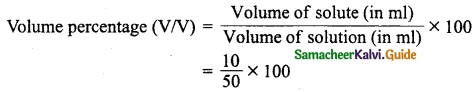= 20% (v/v)

Question 2.
Neomycin, the antibiotic cream contains 300 mg of neomycin sulphate the active ingredient in 30 g of ointment base. Calculate the mass percentage of neomycin.
Mass of neomycin sulphate(solute) = 300 mg
Mass of the ointment (solution) = 30 g
Formula: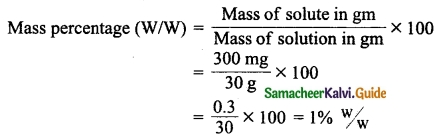VIII. Numerical problems:

Question 1.
Calculate the molality of the solution containing 18 g of Glucose (Molecular mass 180) in 2 kg of water.
Mass of Glucose = 18 g
Molecular mass of Glucose = 180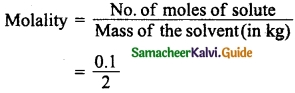= 0. 05 mQuestion 2.
Calculate the molarity of a solution containing 5.85 g of sodium chloride in 500 ml of the water. (Molecular mass = 58.5)
No. of moles of NaCl = $$\frac{5.85}{58.5}$$ = 0.1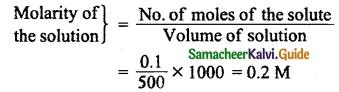= $$\frac{0.1}{500}$$ × 1000 = 0.2 M